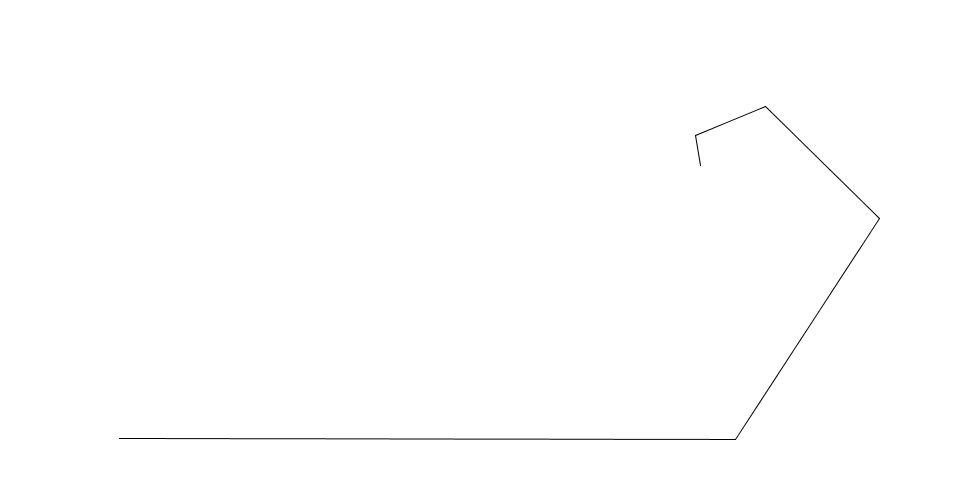# Going Around In A Discrete Harmonic Spiral

Calculus Level 5A man is standing at the origin. First, he walks $1$ unit in the positive $x$ direction and reaches $(1,0)$. Then, he turns $60^o$ counterclockwise and walks $1/2$ units. Again, he turns $60^o$ counterclockwise and walks $1/3$ units. He continues this endlessly.

Find the distance between his initial and final position.

×